Hydraulic Jump

Introduction

A hydraulic jump is a fluid shockwave created at the transition between laminar and turbulent flow. One common example of a hydraulic jump can be seen in the water radiating outward when the stream of tap water strikes the horizontal surface of a sink. The water initially flows in a smooth sheet with consistent current patterns. In this region, the speed of the water exceeds the local wave speed. Friction against the sink surface slows the flow until an abrupt change occurs. At this point, the depth increases as water piles up in the transition region and flow becomes turbulent . The motion of individual water molecules becomes erratic and unpredictable. The interruption of flow patterns also reduces the kinetic energy of the water. In addition to the kitchen sink example, hydraulic jumps are also typical features of river rapids where the water swirls and foams around rocks and logs.

Basic Theory

Although the hydraulic jump effect is common to everyday experience and has been studied experimentally for many years, the underlying theory describing the phenomena is surprisingly complex. Rayleigh first described the problem in 1914 . He calculated of the change in fluid depth associated with the shockwave and introduced the concepts of continuity and fluid momentum conservation in his derivation. A schematic of fluid flow at a hydraulic jump is depicted in Figure 1.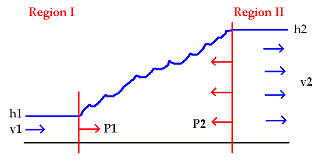Fig. 1: Diagram of classical hydraulic jump.

Let region 1 represent section of fast laminar flow preceding the hydraulic jump and region 2 denote the section of slow turbulent flow after the transition. In the present analysis, assume a uniform hydrostatic pressure distribution and a uniform velocity distribution. Consider vertical slices of fluid representing unit areas of the flow. Continuity of fluid flow implies that that the discharge, q=hv , must be equal before and after the hydraulic jump. h denotes the water depth and v is the water velocity.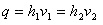Now we can write out the sum of the forces on water between the two regions. Let P=ρgh2/2 represent the pressure force per unit width where ρ is the density of the fluid and g represents gravitational acceleration on the surface of the Earth. If the hydraulic jump occurs over a relatively short distance, the force of friction becomes neglible in the calculation.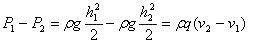A useful quantity to define is the Froude number FR=v/(qh)1/2. The Froude number is the ratio of flow velocity to wave celerity and marks the boundary between critical and subcritical flow . For conditions in which the Froude number is greater than one, flow velocity exceedes the wave celerity (the speed of an individual wave crest) and the fluid motion is smooth. Subcritical currents with Froude numbers less than exhibit turbulent flow.

Next, call the ratio of fluid depths D=h1/h2. Solving the two equations above yields the following differential equation in terms of the ratio of fluid depths and Froude number: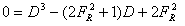The solution of this differential equation provides the ratio of final depth following a hydraulic jump to the initial depth before the jump.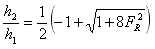Another important property of the hydraulic jump is the energy dissapated by the transition to turbulent flow. The energy loss is usually measured in terms of change in hydraulic head, H=h+(q2/2gA2) where A is the cross sectional area of the flow. Efficiency is written as η=ΔH/H1.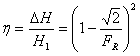Both the change in fluid depth and the energy dissipated can be quantified in terms of the Froude number. As the Froude number increases, the change in fluid depth grows and the energy dissipated by the jump rises.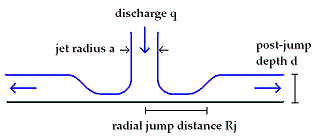Fig. 2: Diagram of radial hydraulic jump

The theoretical framework developed by Rayleigh can be also used to predict the location of a radial hydraulic jump. Consider the arrangement in Figure 2 for a radial hydraulic jump. Following Rayleigh's theoretical approach, one can derive the position of a radial hydraulic jump as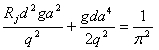Here, Rj is the radial position of the jump, d represents the post-jump depth, a is the jet radius, g is gravitational acceleration, and q is jet discharge. Notice that fluid viscosity does not explicitly enter into Rayleigh's calculation of the jump position in this formula. Indeed, the effect of fluid viscosity on shockwave formation is one of the outstanding theoretical questions concerning hydraulic jumps. In the 1960s, Watson developed a new model to account for the change to turbulent flow following the hydraulic jump . Using a series of approximation techniques, Watson proposed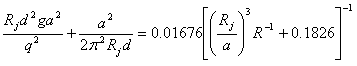with the Reynolds number of the jet defined as R=q/va. The Reynolds number is a dimensionless quantity that provides a scale for conditions of laminar and turbulent flow based upon the fluid properties amd geometry of the system. The Reynolds number can also be thought of as the ratio of inertial forces to viscous forces or as the ratio of the bulk motion of the fluid to smaller scale pertubations such as eddies. A Reynolds number much greater than unity corresponds to turbulent flow while a value comparable or less than one implies that the viscous limit for laminar flow should be applied. Watson's formula can be refined by including an extra factor in first term that accounts for the shape of the jump and fluid surface tension . Bush and Aristoff suggest the following modification to Watson's formula,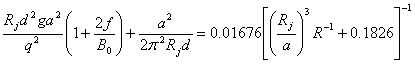where f indicates the sharpness of the transition (ranging from 0 for a smooth change to 1 for an instantaneous jump) and B0 accounts for the surface tension.

Radial Jump for Superfluid Helium

Contemporary experiments have put fluid dynamics models to an extreme test by measuring the radii of hydraulic jumps using liquid helium. Above the lambda point critical temperature, liquid helium behaves as a normal fluid with conventional viscosity properties. However, as the temperature drops below 2.17 K, the liquid helium experiences a phase change and becomes a superfluid with effectively zero viscosity. The vast majority of hydraulic jump models consider conventional fluids and until recently, the ability of these models to describe superfluids was untested. The importance of the hydraulic jumps in the study of fluid dynamics motivates further investigation into the superfluid scenario.

In 2007, Rolley, Guthmann, and Pettersen performed an experiment to analyze radial hydraulic jumps in liquid helium . A jet of liquid helium falls vertically downward onto the surface of a horizontal mirror. The depth of the liquid helium is measured by a CCD camera positioned at a shallow viewing angle. Additional mirrors are positioned at angles to observe the radial position of the jump from above. Next the jet of liquid helium is gradually cooled from an initial temperature of 4.2 K through the superfluid transition point to a final temperature of 1.5 K. The jump radius is measured at liquid helium temperatures above and below the superfluid transition to gauge the effect of fluid viscosity.

Above the critical temperature, the position of the hydraulic jump position is well described by theory as demonstrated by a close correspondence with experimental values. Models not accounting for surface tension tend to overestimate jump radii while models incorporating surface tension tend to predict jump radii slightly too low. More surprisingly though, the model proposed by Watson continued to accurately predict shockwave conditions of liquid helium below 2.17 K. Rolley, Guthmann, and Pettersen explain this effect by observing that the liquid helium below 2.17 K is actually a mixture of normal and superfluid components . Additionally, superfluidity is disrupted above a critical fluid velocity. Therefore, even at a temperature of 1.5 K, the effective viscosity of the liquid helium was certainly non-zero. However, the experimenters were able to confirm the presence of a superfluid component by observing ripple patterns uncharacteristic of normal fluids.

Despite experimental limitations, the accuracy of theoretical predictions of shockwave conditions over a wide range in fluid viscosities indicates that current models may be applicable to a larger range of fluid behavior than previously expected.

Industrial Application as Energy Dissipator

Hydraulic jumps remain a topic of continued scientific and technological interest in part due to their industrial utility as energy dissipators. One of the most important applications of the hydraulic jump is to reduce the impact of dams downstream. Rapid outflow from a spillway erodes the channel and can undermine the structural strength of the reservoir if left unchecked. However, hydraulic jump stilling basins can reduce the discharge energy by up seventy percent . Internal friction and mixing high velocity flow into the larger water volume lowers the speed of outflow. Depending on the type of design, changes in slope, channel width, or obstacles positioned along the spillway trigger the transition to turbulent flow. Forces from hydrostatic uplift, cavitation, vibration, and abrasion create unique engineering challenges for each class of spillway. Consequently, the design of a practical energy dissipator must balance efficiency with durability.

Termination Shock Analog

The termination shock of the Solar System is a more exotic analog of the hydraulic jump. The Sun emits a flow of charged particles traveling outward at speeds of 420 km/s. Along the way, plasma interactions with the interstellar medium slow the particles. The point at which the solar wind drops to a subsonic speed of about 100 km/s in the interstellar medium is called the termination shock. In this region, the solar wind experiences compressional heating, pressure fluctuations, and a sudden magnetic field changes. Beyond the termination shock, the solar wind is effectively stopped by the interstellar medium in a region called the heliosphere. The bow shock enveloping the heliosphere marks the outer edge of the Solar System.

The outer reaches of the Solar System remained largely unexplored until the last decade. Two Voyager Mission spacecraft launched in 1977 offered the best hope of directly probing this region. By 1993, Belcher, Lazarus, McNutt, and Gordon were able to use early observations of the Voyager 1 spacecraft to predict the location of the termination shock . Then in December 2004, Voyager 1 entered a region with high intensities of low-energy (~1 MeV) solar wind particles at a distance of 94 AU from the Sun . The encounter marked the first direct observation of the termination shock. This discovery required the efforts of both Voyager spacecraft simultaneously exploring separate regions of the Solar System. Since the solar wind pressure varies over time due to changing solar activity levels, Voyager 2 was needed as a calibration instrument to distinguish the termination shock from pressure fluctions due to normal solar events. Voyager 2 lags Voyager 1 by about 20 AU in its outward trajectory and the two spacecraft travel in different directions to probe distinct regions of the Solar System boundary. Subsequent observations have revealed that the position of the termination shock shifts by about 10 AU during an eleven-year solar acitivity cycle. Although the plasma interactions involved in the termination shock differ substantially from hydraulic jumps in water, the abrupt transition of fluid behavoir when dropping to subcritical flow velocities is shared by both phenomena.

© 2007 Keith Bechtol. The author grants permission to copy, distribute and display this work in unaltered form, with attributation to the author, for noncommercial purposes only. All other rights, including commercial rights, are reserved to the author.

References

 W. H. Hager, Energy Dissipators and Hydraulic Jump, (Kluwer Academic Publishers, 1992).

 L. Rayleigh, "On the Theory of Long Waves and Bores," Proc. Roy. Soc. Lond. A 90, 324 (1914).

 E. J. Watson, "The spread of a Liquid Jet Over a Horizontal Plane", J. Fluid Mech. 20, 481 (1964).

 E. Rolley, C. Guthmann and M. S. Pettersen, "The Hydraulic Jump and Ripples in Liquid Helium", Physica B: Cond. Mat. 394, 46 (2007).

 R. M. Khatsuria, Hydraulics of Spillways and Energy Dissipators, (Marcel Decker, 2005).

 J. W. Belcher, A. J. Lazarus, R. L. McNutt Jr., and G. S. Gordon, "Solar Wind Conditions in the Outer Heliosphere and the Distance to the Termination Shock", J. Geophys. Res. 98, 177 (1993).

 W. R. Webber, "An Empirical Estimate of the Heliospheric Termination Shock Location with Time with Application to the Intensity Increases of MeV Protons Seen at Voyager 1 in 2002-2005", J. Geophys. Res. 110, 209 (2005).